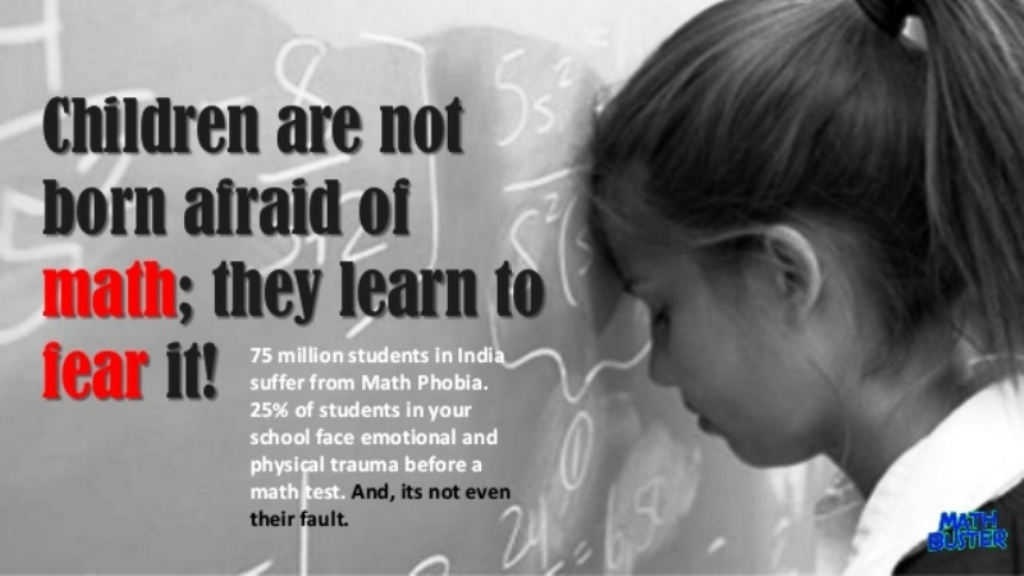Mathematics is very hard for most of the students.  So they avoid topics based on mathematics although there is a hope for propitious future.  For this, it doesn’t help in complaining our children/ students; the fault is in the system of teaching.  There is a huge change in the education system after the arrival of the Mughals and then the Britishers.  This is a unidirectional system.  That’s why there is a problem for students in learning complicated things in a simple way.Mathematics is a vast [multi-functional] subject. There is no subject which is not entailing it. It spreads from our daily business till Astronomy namely Astrology, Cryptography, Musicology, Literature etc., there is a need and it is propagated in all fields. What is the disruption to teach such a great Mathematics according to the rule?

In the Vedic period, India has contributed “0” to the world.  There was a problem for calculating indefinite numbers.  They all were cumbrous.  A “0” resolved all of them.  There are ways to teach such Vedic mathematics as simple and appealing.  But forgetting those is the cause for the problem.

The Primary and secondary educationist Vidya says, there will be 5 sort of students irrespective of classes. They are:

1. Learning through listening
2. Learning through listening and watching
3. Learning from demonstration
4. Group learning
5. Learning by self [personal attention]

Teachers cannot teach by keep in mind of those students in classes.  There the lesson-lecture will be unidirectional.  By this many students are lacking behind in learning.In Singapore, the primary and high school students are in the front line in learning mathematics of same age group to the rest of the countries in the world.  This is because there the syllabus includes Vedic Mathematics and Chinese Mathematical formulas.  Learning is not dry here. If the teaching subject is insignificant, most of the students will be lacking behind in learning.

Vidya assert that,   by studying all these subjects, Nischals learning labs was developed by combining Vedic Mathematics and Chinese Mathematical formulas.  Here the emphasis is given to “see and learn – do and learn” method. This will enable the students of class 1 to 10th to learn in simplex way; what complex the mathematical formulas can be.Vidya insist that if the mathematical formulas are written on the board to teach, only few students will learn.  Many of them do not understand.  By using simple tools of Nischals teaching method, all students will definitely learn. Lot of examples can be given in this regard. If the subject is taught in 5-6 classes, here the students can learn the same in a class- the importance of lab developed in this system is well informed.